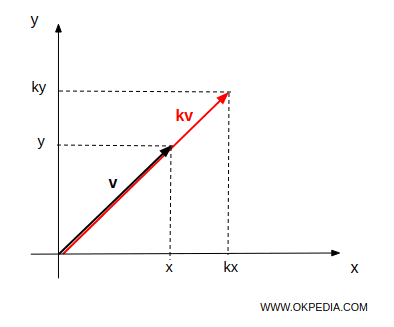# Scalar product

The scalar product of a vector v in Rn by a real number k is a vector u in Rn obtained by multiplying each component of v by k. $$u = v \cdot k = \begin{pmatrix} v_1 \\ v_2 \\ ... \\ v_n \end{pmatrix} \cdot k = \begin{pmatrix} v_1 \cdot k \\ v_2 \cdot k \\ ... \\ v_n \cdot k \end{pmatrix}$$

It is also called a dot product, scalar multiplication or simply product.

If (x, y) is the end point of the vector v, the product kv is a vector with the end point (kx, ky).The product kv of a real number k by vector v is also obtained by multiplying the magnitude of v by k in the same direction if k> 0 or in the opposite direction if k <0.

Example

Given a vector in R3 and a scalar number k = 2

$$u= \begin{pmatrix} 3 \\ 4 \\ 1 \end{pmatrix}$$

The dot product kv is another vector in R3 with each component multiplied by k

$$k \cdot u= 2 \cdot \begin{pmatrix} 3 \\ 4 \\ 1 \end{pmatrix} = \begin{pmatrix} 2 \cdot 3 \\ 2 \cdot 4 \\ 2 \cdot 1 \end{pmatrix} = \begin{pmatrix} 6 \\ 8 \\ 2 \end{pmatrix}$$

www.okpedia.com - Okpedia - Contact email: info@okpedia.it - P.IVA - 09286581005 - Privacy Google - Disclaimer

Vectors

y